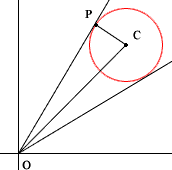Quandaries and Queries Hello, Could you please help me with the following problem: The line with equation y=mx is a tangent to the circle with equation x2+y2-6x-6y+17=0. Find the possible values of m. I am studying in England at A-level which is equivalent, I think, to the last one or two years at high-school. I'm 17, anyway. Thank you, Lech Hi Lech, I completed the squares to put the equation in standard form and got (x-3)2 + (y-3)2 = 1 Hence this is the circle with centre (3,3) and radius 1. The line y=mx is a line through the origin. This information allowed me to draw a diagram and see the two possible tangent lines. On the diagram P is one of the points of tangency, I called it (a,b), C is the centre of the circle (3,3) and O is the origin.Since PO is tangent to the circle, angle CPO is a right angle and thus Pythagoras theorem ensures that |CP|2 + |PO|2 = |OC|2 that is 1 + a2 + b2 = 18 Since P is on the circle, a2 + b2 - 6a -6b + 17 = 0 Substituting from the equation above gives 6a + 6b = 34 or b = 17/3 - a If you now substitute this value for b into a2 + b2 - 6a -6b + 17 = 0 you will have a quadratic in a which you can solve for a. Finally find b from b = 17/3 - a and the unknown slope is b/a Cheers, Harley Go to Math Central